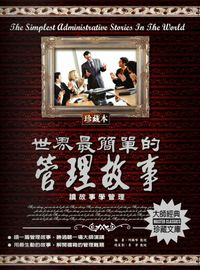### 世界最簡單的管理故事

• 點閱：2
• 作者：
• 出版年：2010[民99]
• 出版社：德威國際文化
• 出版地：臺北市
• 集叢名：一生讀書計畫:54
• ISBN：978-986-6498-70-1 ; 986-6498-70-0

##章節

• 前言(p.5)
• 小鐵錘和大鐵球(p.13)
• 真正的亡羊補牢(p.14)
• 失敗的橫渡海峽(p.16)
• 丟寶石入海(p.17)
• 拴馬的柵欄(p.18)
• 加油站學到的工作態度(p.19)
• 現在正是時候(p.20)
• 借用千斤頂(p.21)
• 品酒大師(p.22)
• 神蹟(p.23)
• 慈善家的轉念(p.24)
• 不要忘記回來(p.25)
• 重複一次別人的話(p.26)
• 教授的實驗(p.28)
• 沙粒和珍珠(p.29)
• 找問題(p.31)
• 致加西亞的信(p.31)
• 一則古希臘神話(p.32)
• 打雜的趙小姐(p.33)
• 道見桑婦(p.34)
• 穿越玉米田(p.35)
• 蜘蛛修網(p.37)
• 半封信的啟示(p.37)
• 偷雞(p.38)
• 紀昌學箭(p.39)
• 純潔的自信心(p.40)
• 鴿子與廣告牌(p.41)
• 買菜的比喻(p.42)
• 蟬與驢(p.42)
• 無欲則剛(p.43)
• 渾沌之死(p.44)
• 不要嚇我(p.45)
• 青蛙王國的國王(p.46)
• 蜈蚣跑腿(p.47)
• 鄰人遺斧(p.48)
• 小宏的褲子(p.49)
• 一個半朋友(p.50)
• 超越百年的後遺症(p.51)
• 齊國弦章拒收賞賜(p.53)
• 千分之一的年薪(p.54)
• 對抗小偷的清潔工(p.55)
• 宋代名臣富弼(p.56)
• 練不完的琴譜(p.57)
• 韓國企業的一日廠長(p.59)
• 日立公司搭鵲橋(p.60)
• 加州的猴子實驗(p.61)
• 堅守晉陽(p.62)
• 何以重用梁丘據(p.63)
• 御史的過失(p.64)
• 特殊的年終獎金(p.65)
• 福特開出的工資(p.67)
• 棋局與戰場的祕密(p.68)
• 哈里遜化解災難(p.69)
• 林肯與陸軍部長(p.70)
• 國亂思良相(p.71)
• 老虎的孤獨(p.73)
• 漢景帝的察人術(p.74)
• 齊桓公用人的成敗之鑑(p.75)
• 曹操和齊高帝的面子(p.77)
• 孫冕罷鹽(p.78)
• 少年丞相(p.79)
• 籠子裏的猴子(p.81)
• 愛炫耀的狐狸(p.82)
• 晏嬰為齊景公創造名望(p.83)
• 司機應該離懸崖多遠(p.84)
• 周亞夫嚴明軍令(p.85)
• 李世民清理吏治(p.86)
• 胡雪巖重金用人(p.88)
• 領頭羊和牧羊犬(p.89)
• 鋸下椅背(p.90)
• V型飛雁(p.91)
• 獻酒(p.92)
• 猴子王國(p.93)
• 小李與科長(p.94)
• 劉秀用降將(p.95)
• 商鞅治秦(p.97)
• 拉車(p.99)
• 兩隻刺蝟(p.100)
• 老農餵牛(p.101)
• 老虎自斷其足(p.101)
• 黃金台招賢(p.102)
• 鯰魚的刺激效應(p.103)
• 從失敗中求勝(p.104)
• 當機不斷(p.105)
• 佛塔上的老鼠(p.106)
• 陶朱公次子之死(p.107)
• 木桶法則(p.109)
• 裴行儉識人(p.109)
• 狩獵的印第安人(p.110)
• 南風法則(p.112)
• 蟻群效應(p.112)
• 才子還是懦夫(p.114)
• 亞歷山大為什麼沒升職(p.115)
• 養蜂的黑熊和棕熊(p.116)
• 第三塊餅(p.118)
• 肯德基的特殊管理(p.119)
• 惠普的周遊式管理(p.119)
• 當有人向你說不時(p.120)
• 黃炳縣令買飯之舉(p.121)
• 日本太陽公司的開會效率(p.122)
• 置身事外的第三者(p.123)
• 分粥的學問(p.124)
• 南隱禪師的一杯茶(p.125)
• 作家明智的選擇(p.126)
• 一杯羹導致敗仗(p.127)
• 子賤放權(p.128)
• 李若谷的修堤管理(p.129)
• 沒有人會死在這裏(p.130)
• 果斷的索羅斯(p.131)
• 驢子和白馬的優勢(p.132)
• 看門的狗(p.133)
• 阿基里斯的弱點(p.133)
• 陸軍與三位連長(p.134)
• 拿破崙救人(p.136)
• 宋太祖誡吏慎刑(p.136)
• 趾高氣揚遭滅亡(p.138)
• 張良以弱制強(p.139)
• 乞丐與狗(p.140)
• 零和遊戲的原理(p.142)
• 一無所知的老海象(p.143)
• 炮兵裏多餘的人(p.145)
• 換一個燈泡需要幾個人(p.146)
• 合作才能雙贏(p.148)
• 如何增加國庫(p.149)
• 福特之意不在酒(p.150)
• 本能(p.151)
• 逆向思考(p.151)
• 制度的力量(p.152)
• 康熙與魏東亭(p.155)
• 潘興將軍的賞識術(p.157)
• 楚王斷帶(p.159)
• 五條毛毛蟲(p.160)
• 五指爭大(p.162)
• 被驅逐的狗(p.164)
• 世界上最好的成功法則(p.165)
• 最短的路未必最快(p.166)
• 跳蚤實驗(p.167)
• 拉上窗簾(p.169)
• 老鼠與米缸(p.170)
• 牛脾氣(p.170)
• 留個缺口給別人(p.172)
• 分工的故事(p.173)
• 標準的故事(p.174)
• 體制的故事(p.175)
• 表率的故事(p.176)
• 溝通的故事(p.177)
• 指導的故事(p.178)
• 鍛鍊的故事(p.179)
• 管仲病榻論相(p.180)
• 愛若和布若(p.181)
• 嚴以律己，寬以待人(p.182)
• 扁鵲的醫術(p.183)
• 野狼磨牙(p.184)
• 曾國藩和賊(p.185)
• 孫權重用諸葛瑾(p.186)
• 年輕人與幸運之神(p.188)
• 青蛙與鮮奶桶(p.190)
• 兔子自殺事件(p.192)
• 一條腿的鴨子(p.193)
• 田穰苴重權立威(p.194)
• 蠍子和青蛙(p.196)
• 樂羊出征(p.198)
• 秦穆公自省(p.199)
• 逆旅二妻(p.201)
• 王琺鑒才(p.202)
• 戴安娜的蘋果(p.203)
• 唐太宗用人(p.204)
• 黃帝與牧童(p.206)
• 批評的藝術(p.207)
• 和尚爭功(p.209)
• 竊賊的長處(p.210)
• 醒悟的猴子(p.211)
• 猴子與鐘錶(p.214)
• 墨子責徒(p.216)
• 煮雞蛋的技巧(p.217)
• 小金魚的報復(p.218)
• 離家出走的狗(p.220)
• 忘恩的雇主(p.222)
• 莊子釋領導之道(p.223)
• 複雜管理的背後(p.224)
• 宰相剃頭(p.226)
• 宋太宗醉酒(p.228)
• 蘇掖買屋(p.229)
• 海島的國王(p.230)
• 老鷹和鴨子(p.231)
• 討厭你，但我要提拔你(p.232)
• 問時間(p.233)
• 繩子牽羊(p.234)
• 媳婦和頑石(p.235)
• 小馬逃跑的故事(p.236)
• 茉莉香歌舞團(p.237)
• 幸福的小狐狸(p.238)
• 胡雪巖攬才(p.240)
• 李世民的吏治(p.241)
• 鞋匠的兒子(p.243)
• 十羊九牧(p.244)
• 楚國大將(p.245)
• 誰來管倉庫(p.246)
• 劉備延攬徐庶(p.248)
• 螞蟻的知識管理(p.249)
• 不知趣的烏龜(p.250)
• 螃蟹文化(p.252)
• 金別針與激勵(p.253)
• 小黑羊救命(p.254)
• 野羊的選擇(p.255)
• 太子割羊肉(p.256)
• 猿猴與蜈蚣(p.257)
• 小女孩學跳舞(p.258)
• 狗熊救火(p.259)
• 鼴鼠的忠告(p.260)
• 貓頭鷹飼養老鼠(p.261)
• 虛偽的喜鵲(p.262)
• 雙頭鳥(p.263)
• 吃西瓜(p.264)
• 慷慨的農夫(p.265)
• 薔薇與雞冠花(p.266)
• 韓信點兵(p.267)
• 職場的狗和貓(p.268)
• 倖存的猶太傳教士(p.269)
• 一只箱子(p.270)
• 重新認識一個人(p.272)
• 一百元到一百億元(p.273)
• 堅持就是勝利(p.274)
• 旋轉的門(p.275)
• 生命之樹也有落葉(p.276)
• 工作的價值(p.277)
• 熱情不會浪費(p.278)
• 老虎和牛虻(p.279)
• 把眼光放遠一點(p.280)
• 大學圓夢(p.281)
• 智慧是等不來的(p.282)
• 漁王的兒子(p.283)
• 成為一顆珍珠(p.284)
• 逃避不是辦法(p.285)
• 永遠不會有孩子(p.286)
• 成功的腳印(p.287)
• 幸福在哪裡(p.288)
• 輸得有道理(p.289)
• 飛馬騰空(p.290)
• 貓兒守廚房(p.291)
• 溫暖的衝動(p.293)
• 孔子懷疑徒弟(p.293)
• 「一滴智慧」造就石油大王(p.294)
• 一百美元借款(p.295)
• 最堅固的門(p.297)
• 修路原則(p.298)
• 災後總結(p.299)

##同作者書籍世界最偉大的品格書:...